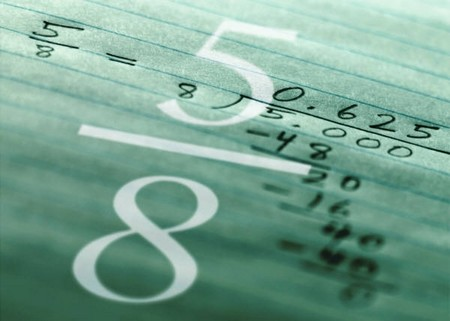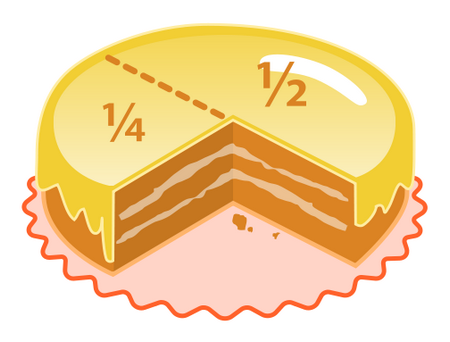Multiplying and dividing fractions are easier as everyone says, but what everyone hates is adding or subtracting them. Addition is the basic arithmetic operation and no one can do without it. However hard one tries to avoid them there is just no escape route. Fractions are an important part of the education of any child. If the fundamentals are not cleared properly the child may not be able to do well in mathematics as fractions are an integral part of the number system. You may hire someone to provide math tutoring to further help your child understand fractions and all the other math topics he/she is having difficulties with.

Items Needed:

• Pen
• PaperStep 1

Remember that a fraction is divided in two parts; the numerator and the denominator. The number on the top is called the numerator and the one at the bottom is known as the denominator.

Step 2

Get the same value for the denominator. The main thinning to be done is to get the same value for all the denominators of the fractions you are adding. One of the easiest ways is to multiply the second denominator with that of the first and vice versa. Remember you also have to multiply the numerator by the same number by which you multiplied the denominator. If the denominator is multiplied by 7 also multiply the numerator by 7, else the value of the fraction will change. For more than two fractions one can take the Lowest Common Multiple of the denominators.

Step 3

Add the numerators once you get the same denominator for all the fractions. Keep the same denominator for the fraction.

Step 4

Reduce the fraction to the lowest terms. A fraction should always be represented in the lowest terms. This means that both the numerator and the denominator should be divided by the same number till the point that it cannot be reduced any further by the same number.Tip:

The main goal is to make the denominator the same for all the fractions that have to be added.

Warning:

If the denominators are not the same the fractions cannot be added so make sure that you use any method and make the denominator the same for all fractions. If the fraction is not reduced to the lowest terms the answer will be wrong and not accepted so ensure that the fraction is presented in the simplest terms.

Filed Under: Education & Training

Tags:About the Author: Darlene Aronson holds a degree in English literature and is a college teacher in Texas, USA. She likes to help others by sharing her experiences in education and training field. She has written for many blogs as well as local magazines.